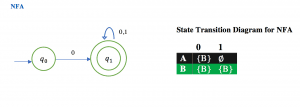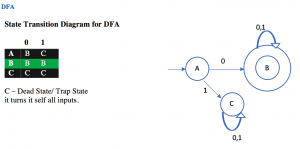## Example of Conversion of Non-Deterministic Finite Automata (NFA) to Deterministic Finite Automata (DFA) – 5

Example : Design a NFA for a language that accepts all string over {0,1}, in which the second last symbol is always 1. Then convert it to its equivalent DFA.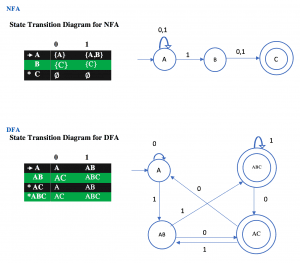## Example of Conversion of Non-Deterministic Finite Automata (NFA) to Deterministic Finite Automata (DFA) – 4

Example :Given below is the NFA for a language L= {Set of all string over (0, 1) that ends with 01 }  construct its equivalent DFA.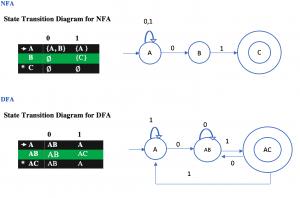## Example of Conversion of Non-Deterministic Finite Automata (NFA) to Deterministic Finite Automata (DFA) – 3

Example:  Find the equivalent DFA for the given by M= [{A, B, C}, (a, b), , A, {C}] where δ is given by: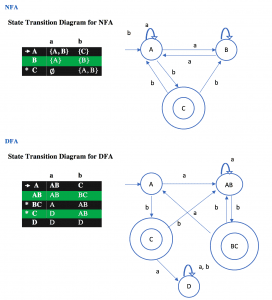## Example of Conversion of Non-Deterministic Finite Automata (NFA) to Deterministic Finite Automata (DFA) – 2

Example :  L = {Set of all string over (0,1) that ends with 1}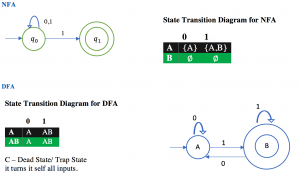## Example of Conversion of Non-Deterministic Finite Automata (NFA) to Deterministic Finite Automata (DFA) – 1

Example:  L= {Set of all strings over (0,1) that start with 0}